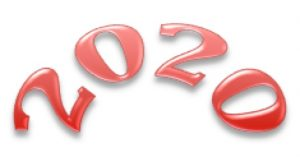Is it correct to say “twenty twenty” or should we say “two thousand and twenty” or are both correct?  Why?

People say “twenty twenty” so do the 2 twenties mean – 20 thousands, 20 hundreds, 20 tens or 20 units?

You can be a creative mathematician, you can be someone who has your own mathematical ideas.

Find your own interesting facts about 2020 and calculations that have the answer 2020. For example can you find factors of 2020?

You might think of calculations like: $101 \times 20 = 2020$ or more complicated ones … for example $2^{11} – 3^{3} – 1= 2020$ or the sum of four consecutive primes $17^2 + 19^2 + 23^2 +29^2$. You might like to use a calculator.

What different questions can you find with 2020 as the answer?

Compare your questions with other people’s.

Perhaps you can make up one that is easy, one that is harder and one that is very hard.

What interesting facts can you find about the year that you were born?